## Student Chemical Equations PDF Details

As a student, you are likely to come across chemical equations in your coursework. These equations can be daunting, but with the right approach, they can be easy to understand and work with. In this blog post, we'll take a look at the different parts of a chemical equation and how to use them. We'll also provide some tips for working with these equations.

You might find it helpful to know the amount of time you'll need to fill out this student chemical equations and how lengthy this form is.

Form NameStudent Chemical Equations
Form Length5 pages
Fillable?Yes
Fillable fields79
Avg. time to fill out17 min 3 sec

## Form Preview Example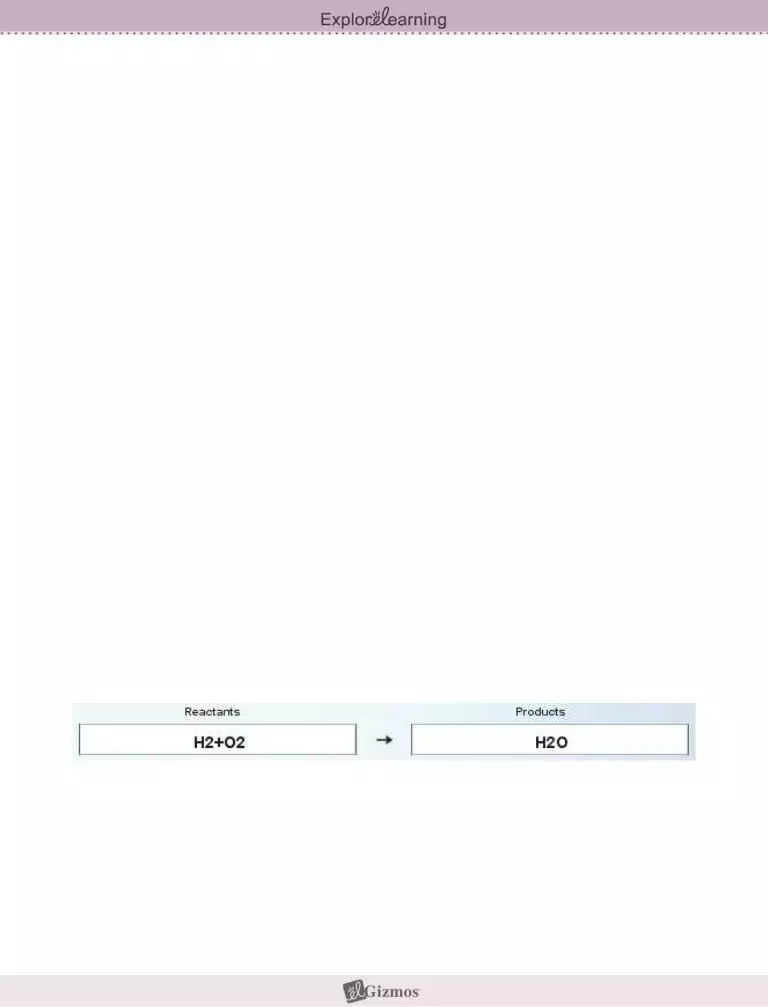Name: ______________________________________ Date: ________________________

Student Exploration: Chemical Equations

Vocabulary: Avogadro’s number, chemical equation, chemical formula, chemical reaction, coefficient, combination, combustion, conservation of matter, decomposition, double replacement, molar mass, mole, molecule, product, reactant, single replacement, subscript

Prior Knowledge Questions (Do these BEFORE using the Gizmo.)

1.A candle is placed on one pan of a balance, and an equal weight is placed on the other pan. What would happen if you lit up the candle and waited for a while? ____________________

_________________________________________________________________________

2.Suppose the candle was placed in a large, sealed jar that allowed it to burn for several minutes before running out of oxygen. The candle and jar are balanced by an equal weight.

In this situation, what would happen if you lit up the candle and waited? ________________

_________________________________________________________________________

Gizmo Warm-up

Burning is an example of a chemical reaction. The law of conservation of matter states that no atoms are created or destroyed in a chemical reaction. Therefore, a balanced chemical equation will show the same number of each type of atom on each side of the equation.

To set up an equation in the Chemical Equations Gizmo™, type the chemical formulas into the text boxes of the Gizmo. First, type in “H2+O2” in the Reactants box and “H2O” in the Products box. This represents the reaction of hydrogen and oxygen gas to form water.

1. Check that the Visual display is chosen on each side of the Gizmo, and count the atoms.

A. How many hydrogen atoms are on the Reactants side? ____ Products side? ____

B. How many oxygen atoms are on the Reactants side? ____ Products side? ____

2. Based on what you see, is this equation currently balanced? _________________________Activity A:

Interpreting chemical formulas

Erase the chemical formulas in each text box.

Check that the Visual displays are selected.

Introduction: To balance a chemical equation, you first need to be able to count how many atoms of each element are on each side of the equation. In this activity, you will practice counting the atoms that are represented in chemical formulas.

Question: How do we read chemical formulas?

1.Observe: Type “H2” into the Reactants box and hit Enter on your keyboard. Note that the formula is shown as H2 below. The small “2” in H2 is a subscript.

A.What does the “2” in H2 represent? _______________________________________

B.In general, what do you think a subscript in a chemical formula tells you? _________

___________________________________________________________________

C.Try typing in other subscripts next to the H, such as 3, 4, and 5. Is your answer to question B still true? Explain. ____________________________________________

2.Count: Clear the Reactants box, and type in a more complex chemical formula: “Ca(OH)2.” Look at the number of atoms shown.

A. How many of each type of atom do you see? Ca: _____ O: _____ H: _____

B.In general, what happens when a subscript is found outside of parentheses?

___________________________________________________________________

C.Try typing in other subscripts next to the (OH), such as 3, 4, and 5. Is your answer to question B still true? Explain. ____________________________________________

3.Practice: For each of the real chemical formulas below, calculate how many of each element there are. Check your answers for the first three formulas using the Gizmo.

 AgCl3Cu2 Ag: _____ Cl: _____ Cu: _____ Ba(AsO4)2 Ba: _____ As: _____ O: _____ (NH4)3PO4 N: _____ H: _____ P: _____ O: _____ MnPb8(Si2O7)3 Mn: _____ Pb: _____ Si: _____ O: _____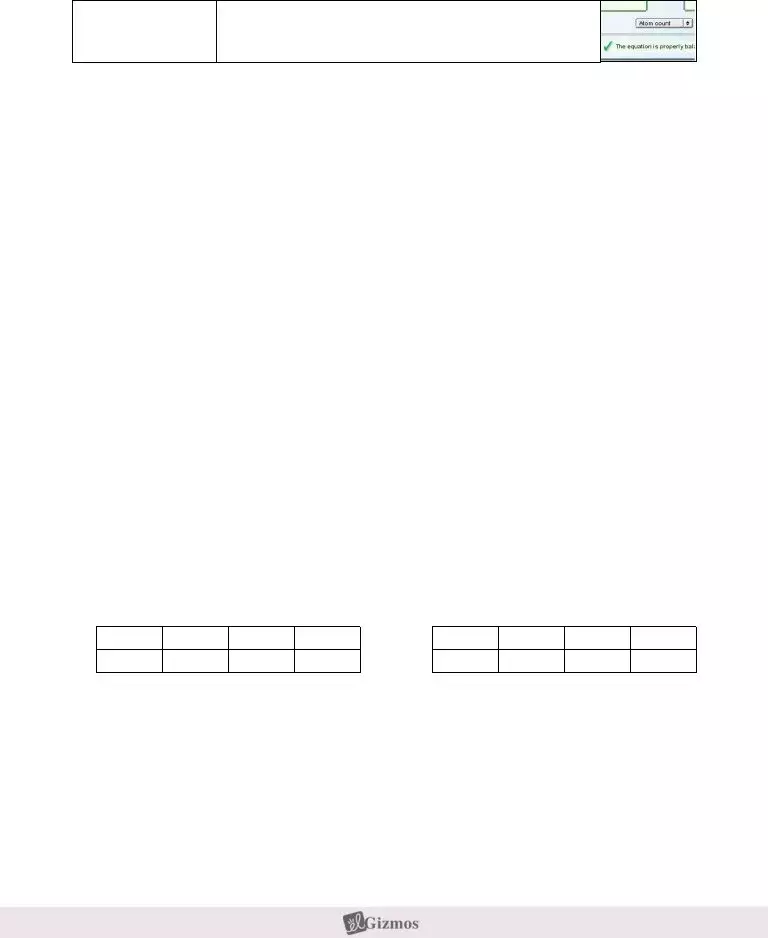Activity B:

Balancing equations

Erase the chemical formulas in each text box.

Introduction: In a chemical reaction, the reactants are the substances that enter into the reaction, and the products are the substances that are made in the reaction. A chemical reaction is balanced if the numbers of reactant atoms match the numbers of product atoms.

Goal: Learn to balance any chemical equation.

1.Observe: To model how hydrogen and oxygen react to make water, type “H2+O2” into the Reactants box and “H2O” into the Products box.

As the equation is written, which element is not in balance? ________________________

Explain: _________________________________________________________________

2.Balance: To balance a chemical equation, you are not allowed to change the chemical formulas of the substances involved in the reaction. You are allowed to change the number of molecules of each substance by adding coefficients in front of the formulas.

A.To balance the oxygen atoms, add a “2” in front of the “H2O” in the Products box. How many oxygen atoms are found on each side of the equation now? _________

B.To balance the hydrogen atoms, add a “2” in front of the “H2” in the Reactants box. How many hydrogen atoms are found on each side of the equation now? _________

C.Is this equation currently balanced? _________ Click Show if balanced to check.

3.Apply: Now enter a more complex chemical reaction: Ca(OH)2 + HBr CaBr2 + H2O. List the numbers of each element in the tables below:

Ca

Reactants

OH

Br

Ca

Products

OH

Br

A.Which elements are out of balance? ______________________________________

B.Add coefficients to balance first the bromine (Br) and then the hydrogen (H) atoms. When the equation is balanced, write the complete formula below:

___________________________________________________________________

(Activity B continued on next page)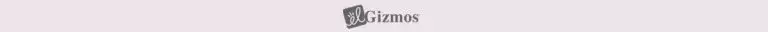Activity B (continued from previous page)

4.Practice: Chemical reactions are generally classified into five groups, defined below. Balance each equation, using the Gizmo for help.

Combination (or synthesis) – two or more elements combine to form a compound.

 • Na + O2 Na2O _________________________________________ • La2O3 + H2O La(OH)3 _________________________________________ • N2O5 + H2O HNO3 _________________________________________ Decomposition – a compound breaks down into elements and/or simpler compounds. • KNO3 KNO2 + O2 _________________________________________ • NaN3 Na + N2 _________________________________________ • NH4NO3 N2O + H2O _________________________________________ Combustion – a fuel reacts with oxygen to release carbon dioxide, water, and heat. • CH4 + O2 CO2 + H2O _________________________________________ • C3H8 + O2 CO2 + H2O _________________________________________

C6H12O6 + O2 CO2 + H2O _________________________________________

Single replacement – an element replaces another element in a compound.

 • KCl + F2 KF + Cl2 _________________________________________ • Mg + HCl MgCl2 + H2 _________________________________________ • Cu + AgNO3 Cu(NO3)2 + Ag _________________________________________ Double replacement – two compounds switch parts with one another. • AgNO3 + K2SO4 Ag2SO4 + KNO3 ___________________________________ • Mg(OH)2 + HCl MgCl2 + H2O ___________________________________

Al(OH)3 + H2SO4 Al2(SO4)3 + H2O ___________________________________Activity C: Molar mass

Erase the chemical formulas in each text box.

In the middle menu, select Molar mass.

Introduction: Chemists are often interested in obtaining a certain amount of product from a chemical reaction. But how is this done? To calculate the proportions of reactants needed to form a desired product, it is necessary to understand a unit of quantity called the mole.

Question: How do chemists know how much of each substance to mix?

1.Observe: The masses of atoms and molecules are measured in universal mass units (u). A universal mass unit is approximately the mass of a proton. Hydrogen gas has a molecular mass of 2.0158 u.

A.Type the formula “H2” into the Reactants box. What is the molar mass of hydrogen gas, H2? ________________________

B.What is the relationship between the molecular mass and the molar mass of a substance? _________________________________________________________

The molar mass of a substance is the mass of one mole of the substance. There are 6.0221415 × 1023 molecules (or atoms) of a substance in one mole. (This value is called Avogadro’s number.)

2.Gather data: The balanced equation to synthesize water is: 2H2 + O2 2H2O. Use the Gizmo to find the molar masses of each substance in this equation:

 2H2 __________ O2 __________ 2H2O __________

3.Analyze: Based on the molar masses, how can you tell that an equation is balanced?

_________________________________________________________________________

_________________________________________________________________________

4.Challenge yourself: Suppose you wanted to make 100 grams of water. How much hydrogen and oxygen would you need to make 100 grams of water with nothing left over? Explain your answer.

Hydrogen: __________Oxygen: __________

_________________________________________________________________________

_________________________________________________________________________

_________________________________________________________________________

Our top level web developers have worked collectively to make the PDF editor that you'll operate. The following software allows you to fill in chemical changes gizmo answers pdf documents quickly and efficiently. This is all you need to do.

Step 1: Choose the "Get Form Now" button to begin the process.

Step 2: Now you may edit the chemical changes gizmo answers pdf. You may use the multifunctional toolbar to insert, eliminate, and transform the content of the file.

Enter the information required by the application to complete the file.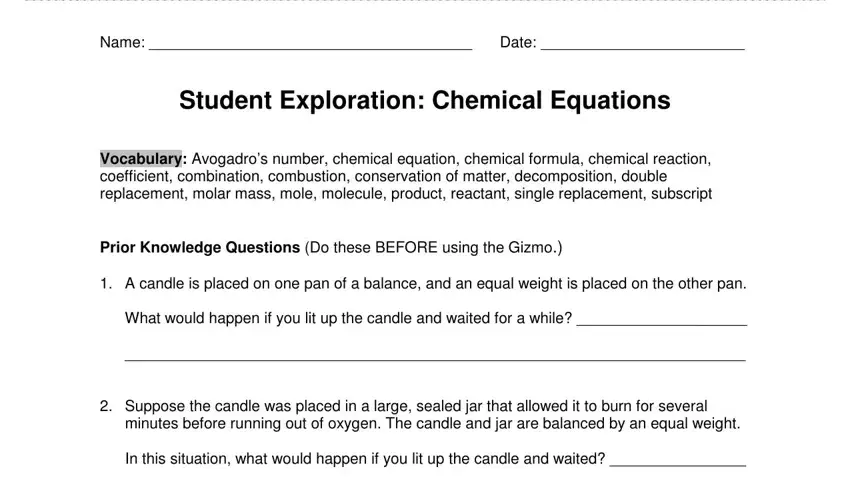Type in the necessary details in the area .The software will request information to automatically fill out the box Get, the, Gizmo, ready and A, What, does, the, in, H, represent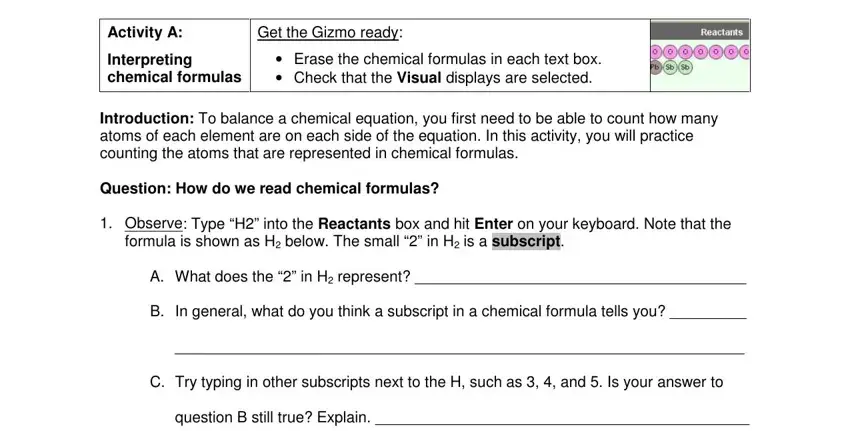When it comes to space Look, at, the, number, of, atoms, shown question, B, still, true, Explain and AgCl, CuBa, As, ON, HPO, M, nPb, SiO define the rights and obligations.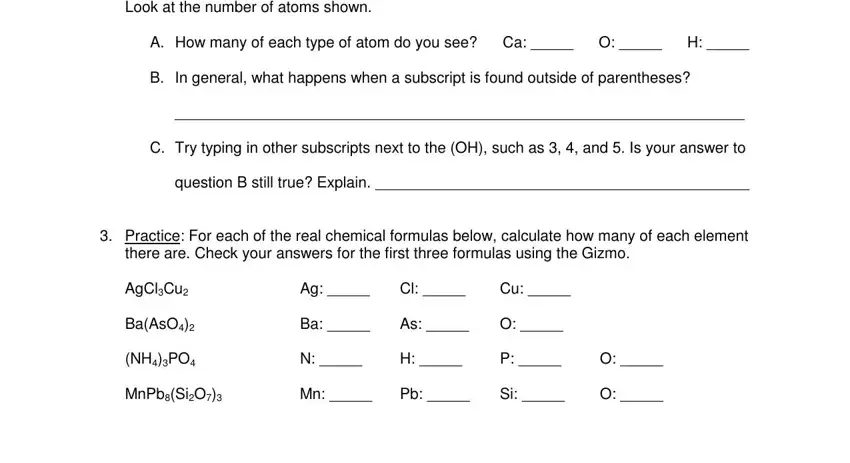Review the sections Activity, B, Balancing, equations Get, the, Gizmo, ready and Reactants, box, and, HO, into, the, Products, box and next fill them out.Step 3: Select the button "Done". The PDF form may be exported. You can easily download it to your device or send it by email.

Step 4: In order to avoid any complications down the road, be sure to prepare up to a few duplicates of your file.